# Week 6 - Chemistry Flashcards

Set Details Share
created 3 years ago by SkyRose
3,209 views
Subjects:
chemistry
Page to share:
Embed this setcancel
COPY
code changes based on your size selection
Size:
X

1

What are the reactants in the following equation: HCl(aq) + NaHCO₃(aq)→ CO₂(g) + H₂O(l) + NaCl(aq)

A) HCl, NaHCO₃

B) HCl, CO₂

C) NaHCO₃, H₂O

D) CO₂, H₂O

E) H₂O, NaCl, CO₂

A) HCl, NaHCO₃

2

Balance the following chemical reaction:

Al₂(SO₄)₃ (aq) + K₃PO₄ (aq) → AlPO₄ (s) + K₂SO₄ (aq)

A) Al₂(SO₄)₃ (aq) + 2 K₃PO₄ (aq) → 2 AlPO₄ (s) + 3 K₂SO₄ (aq)

B) 2 Al₂(SO₄)₃ (aq) + 6 K₃PO₄ (aq) → 3 AlPO₄ (s) + 6 K₂SO₄ (aq)

C) 2 Al₂(SO₄)₃ (aq) + 4 K₃PO₄ (aq) → 4 AlPO₄ (s) + 6 K₂SO₄ (aq)

D) AlSO₄ (aq) + KPO₄ (aq) → AlPO₄ (s) + KSO₄ (aq)+

A) Al₂(SO₄)₃ (aq) + 2 K₃PO₄ (aq) → 2 AlPO₄ (s) + 3 K₂SO₄ (aq)

3

How many oxygen atoms are located on the reactant side of the following chemical equation?

2 Al(s) + Fe₂O₃(s) → Al₂O₃(s) + 2 Fe(l)

A) 1

B) 2

C) 3

D) 4

E) 5

C) 3

4

What numbers belong in the blank spaces to fully balance the following reaction? __Fe (s) + __Cl₂ (g) → __FeCl₃ (s)

A) 1,1,1

B) 1,2,1

C) 2,3,2

D) 2,2,2

E) 3,3,2

C) 2,3,2

5

When propanol (C₃H₇OH) is burned in excess oxygen, what is/are the product(s) of this reaction?

A) CO₂

B) H₂O, O₂

C) H₂O

D) CO₂, H₂O

D) CO₂, H₂O

6

When butanol (C₄H₉OH) is burned in oxygen, CO₂ and H₂O are the products. What is the coefficient in front of the O₂ in the balanced equation (using only whole numbers and the lowest whole number ratio)?

6

7

What are the coefficients in front of the H₂ and the Au if you balance the following unbalanced equation: Au₂S₃ + H₂ → Au + H₂S?

A) 3, 6

B) 6, 4

C) 6, 2

D) 3, 2

D) 3, 2

8

What is the coefficient in front of the O₂ when the equation below is balanced (using only whole numbers and the lowest whole number ratio)? C₂H₄(g) + O₂ (g) → CO₂(g) + H₂O (g)

3

9

Give the balanced equation for solid iron(III) sulfide reacting with gaseous hydrogen chloride to form solid iron(III) chloride and hydrogen sulfide gas.

A) Fe₂S₃ (s) + HCl (g) → FeCl₃ (s) + H₂S (g)

B) Fe₂(SO₄)₃ (s) + 6 HCl (g) → 2 FeCl₃ (s) + 3 H₂S (g)

C) Fe₂S₃ (s) + 6 HCl (g) → 2 FeCl₃ (s) + 3 H₂S (g)

D) Fe₃S (s) + HCl (g) → Fe₃Cl (s) + H₂S (g)

C) Fe₂S₃ (s) + 6 HCl (g) → 2 FeCl₃ (s) + 3 H₂S (g)

10

Balance the following chemical equation (if necessary) for the combustion reaction of glucose:

C₆H₁₂O₆(s) + O₂(g) → CO₂(g) + H₂O(g)

C₆H₁₂O₆(s) + 6O₂(g) → 6CO₂(g) + 6H₂O(g)

11

Balance the following chemical equation (if necessary):

Ca(C₂H₃O₂)₂(aq) + Na₂CO₃(aq) → CaCO₃(s) + NaC₂H₃O₂(aq)

Ca(C₂H₃O₂)₂(aq) + Na₂CO₃(aq) → CaCO₃(s) + 2 NaC₂H₃O₂(aq)

12

Balance the following chemical equation (if necessary):

SiCl₄(s) + H₂O(l) → H₄SiO₄(aq) + HCl(aq)

SiCl₄(s) + 4 H₂O(l) → H₄SiO₄(aq) + 4 HCl(aq)

13

Balance the following chemical equation (if necessary):

NaOH(aq) + H₂SO₄(aq) → H₂O(l) + Na₂SO₄(aq)

2NaOH(aq) + H₂SO₄(aq) → 2H₂O(l) + Na₂SO₄(aq)

14

Balance the following chemical equation (if necessary):

Na⁺(aq) + Cl⁻(aq) + Ag⁺(aq) + NO₃⁻(aq) → Na⁺(aq) + AgCl(s) + NO₃⁻(aq)

Na⁺(aq) + Cl⁻(aq) + Ag⁺(aq) + NO₃⁻(aq) → Na⁺(aq) + AgCl(s) + NO₃⁻(aq)

15

Balance the following chemical equation (if necessary):

MnO₂(s) + H₂O(l) + Zn(s) → Mn(OH)₂(s) + Zn(OH)₂(s)

MnO₂(s) + 2 H₂O(l) + Zn(s) → Mn(OH)₂(s) + Zn(OH)₂(s)

16

Balance the following chemical equation (if necessary):

I₂(s) + 2Na₂S₂O₃(aq) → Na₂S₄O₆(aq) + NaI(aq)

I₂(s) + 2Na₂S₂O₃(aq) → Na₂S₄O₆(aq) + 2NaI(aq)

17

Write a balanced chemical equation based on the following description: solid chromium reacts with solid iodine to form solid chromium(III) iodide.

2 Cr(s) + 3 I₂(s) → 2 CrI₃(s)

18

Write a balanced chemical equation based on the following description: butanoic acid, C₃H₇COOH(l) burns in air

C₃H₇COOH(l) + 5 O₂(g) → 4 CO₂(g) + 4 H₂O(g)

19

Write a balanced chemical equation based on the following description: solid magnesium metal reacts with chlorine gas to produce solid magnesium chloride

Mg(s) + Cl₂(g) → MgCl₂(s)

20

Write a balanced chemical equation based on the following description: solid calcium carbonate decomposes into solid calcium oxide and carbon dioxide gas.

CaCO₃(s) → CaO(s) + CO₂(g)

21

Which of the following represents the generic form of a double-displacement reaction?

A) A + B → AB

B) A + BX → AX + B

C) AX + BY → AY + BX

D) AB → A + B

C) AX + BY → AY + BX

22

What type of reaction is represented by the following equation: 2Na(s) + Cl₂(g) → 2NaCl(s)

A) combination

B) decomposition

C) double displacement

D) single displacement

A) combination

23

What type of reaction is represented by the following equation: BaCl₂(aq) + Na₂SO₄(aq) → BaSO₄(s) + 2NaCl(aq)

A) combination

B) decomposition

C) double displacement

D) single displacement

C) double displacement

24

What type of reaction is represented by the following equation: C₄H₈(g) + 6O₂(g) → 4CO₂(g) + 4H₂O(g)

A) acid-base

B) decomposition

C) precipitation

D) redox (oxidation-reduction)

D) redox (oxidation-reduction)

25

What type of reaction is represented by the following equation: SiCl₄(l) + 2Mg(s) → 2MgCl₂(s) + Si(s)

A) combination

B) decomposition

C) double displacement

D) single displacement

D) single displacement

26

In the following reaction, which element in what species is oxidized? Au(NO₃)₃ (aq) + 3 Li(s) → Au(s) + 3 LiNO₃ (aq)

A) Au in Au(NO₃)₃

B) N in Au(NO₃)₃

C) O in Au(NO₃)₃

D) Li

E) This is not an oxidation/reduction type of reaction.

D) Li

27

In the following reaction, which element in what species is reduced? Zn (s) + 2 HCl (aq) → ZnCl₂ (aq) + H₂ (g)

A) Zn

B) H in HCl

C) Cl in HCl

D) This is not an oxidation/reduction type of reaction.+

B) H in HCl

28

In the following reaction, which element in what species is reduced? NaOH (aq) + HCl (aq) → NaCl (aq) + H₂O(l)

A) Na in NaOH

B) O in NaOH

C) H in HCl

D) Cl in HCl

E) This is not an oxidation/reduction type of reaction.

E) This is not an oxidation/reduction type of reaction.

29

Which of the following contains the highest number of atoms?

A) 1 mole of helium

B) 1 mole of carbon

C) 1 mole of uranium

D) All contain an equal number of atoms

E) Not enough info

D) All contain an equal number of atoms

30

Fill in the blank: A mole is defined as the number of carbon atoms in __________ g of pure ¹²C.

12

31

What is the mass of one mole of carbon atoms?

12.01

32

Determine the mass in grams of 3.00 × 10²¹ atoms of arsenic. (The mass of one mole of arsenic is 74.92 g.)

0.373 g

33

What is the value of Avogadro's number?

6.022 × 10²³

34

Calculate the mass, in grams, of 202 atoms of iron, Fe (1 mol of Fe has a mass of 55.85 g).

1.87 × 10⁻²⁰ g

35

Determine the number of atoms in 30.0 g of K.

4.62 × 10²³ atoms

36

Determine the mass in grams of 1.6 × 10⁻³mol glucose, C₆H₁₂O₆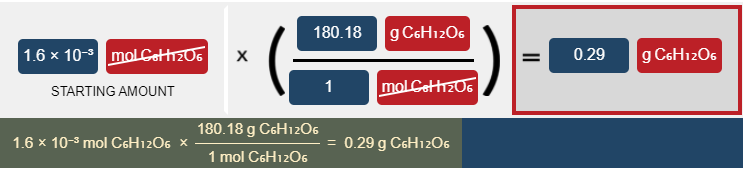0.29 g C₆H₁₂O₆.

37

Determine the number of moles in 4.21 × 10²³molecules of CaCl₂4.21 × 10²³moleculesCaCl₂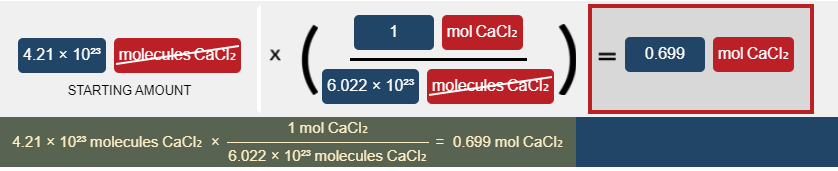0.699 mol CaCl₂.

38

How many moles of propylene (C₃H₆) are in 25.0g of the substance?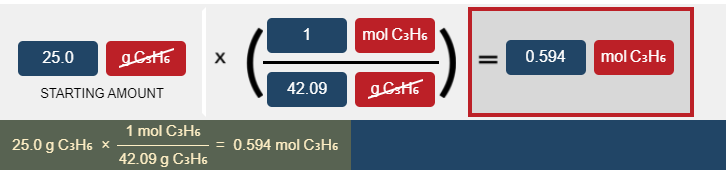0.594 mol C₃H₆.

39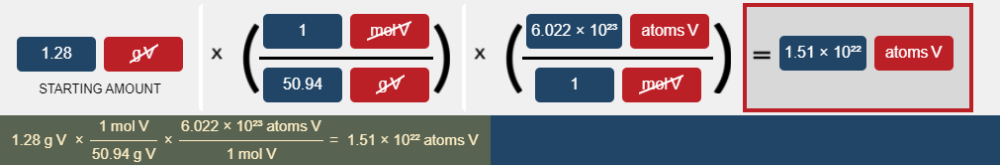1.51 × 10²² atoms V.

40

How many grams are in 5.32 × 10²² molecules of CO₂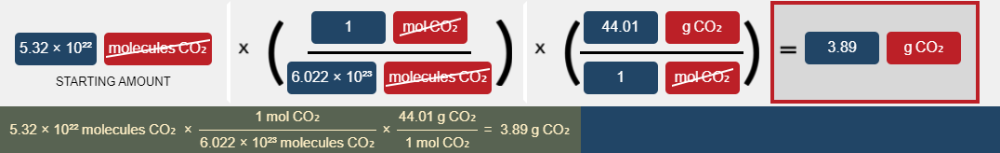3.89 g CO₂

41

To convert from grams to number of molecules, you need to use:

B) Molar mass

C) Density

D) Both (A) and (B)

E) None of the above

D) Both (A) and (B)

42

Which one of the following contains the most moles of oxygen atoms?

A) 1 mol of Fe(C₂H₃O₂)₃

B) 1 mol of Na₃PO₄

C) 2 mol of Ca(NO₂)₂

D) 3 mol of CH₃OH

C) 2 mol of Ca(NO₂)₂

43

How many moles of RbF are in 57.0 grams of RbF?

0.546 mol

44

If 4.9 kg of CO₂ are produced during a combustion reaction, how many molecules of CO₂ would be produced?

6.7 × 10²⁵ molecules

45

Determine the number of atoms of O in 92.3 moles of Cr₃(PO₄)₂.

4.45 × 10²⁶ atoms

46

According to the balanced reaction below, calculate the moles of NH₃ that form when 4.2mol of N₂H₄ completely reacts 3N₂H₄(l)→4NH₃(g)+N₂(g)4.2molN₂H₄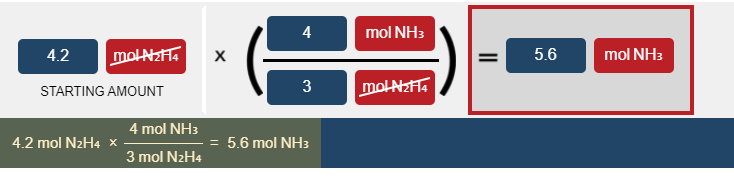5.6 mol NH₃.

47

How many moles of C are formed upon complete reaction of 2mol of B according to the generic chemical reaction: A+2B→C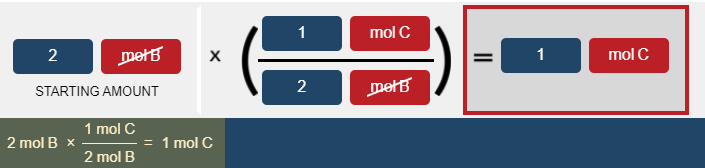1 mol C.

48

How many moles of H₂SO₄ are required to completely react with 7.20mol of Al according to the balanced chemical reaction: 2Al(s)+3H₂SO₄(aq)→Al₂(SO₄)₃(aq)+3H₂(g)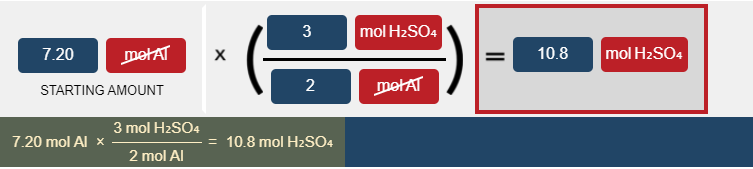10.8 mol H₂SO₄.